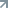# Escalation protocol

This topic was edited by a BMC Contributor and has not been approved.  More information.

BMC's support teams strive to resolve your issues and service requests as quickly as possible based on incident severity level. If you are not satisfied with the progress of your request, you may request an escalation. When you invoke the escalation process, additional levels of management and technical resources may be assigned to help in the prioritization and resolution of the request. BMC asks that you follow, in the order listed, the defined escalation process:

1. Contact the Technical Support Analyst by creating a new Case in Support Central. Alternatively, you may call BMC Support, reference your ticket number, and ask to speak to the on-call duty manager.
2. Contact your Customer Success Specialist.
3. Contact your BMC Account Manager.

Submitting... Thank you

1.Mahesh Yanamalamanda

Change Request CRQ000001318065

Take a backup of table T5445

• Run below query to update order status from DB

update T5445 set c303411200 = 'Handover' where 1="000000000005043" AND 1="000000000120729" AND 1="000000000144460" AND 1="000000000150875" AND 1="000000000238276" AND 1="000000000238865" AND 1="000000000241355" AND 1="000000000247074" AND 1="000000000247907" AND 1="000000000249324" AND 1="000000000250792" AND 1="000000000253392" AND 1="000000000253399" AND 1="000000000253411" AND 1="000000000255078" AND 1="000000000258467" AND 1="000000000259160" AND 1="000000000259303" AND 1="000000000259596" AND 1="000000000261714" AND 1="000000000262066" AND 1="000000000262193"

These records are in the archive, so we are asked to submit the above change request to change to Handover, but not a single record has changed.

Can you please provide the escalation process and contact numbers?

Aug 21, 2023 05:29
1.Dhanya Menon

Hello Mahesh,